1.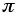2. f(x) = x18-12x15+36x12-2560x9-13104x6-17472x3-64 is one such function.
.......Update: Acknowledgements to correspondent Jeremy Weissmann, who found the following polynomial of degree 6:
.......f(x) = x6-6x4-4x3+12x2-24x-4 .

3. s = 35 ; n = 4 ; k = 9

4.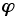, or phi, also known as the golden ratio or golden section. It is equal to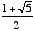.

5. (sinh 1)/2

6. (sinh 1 - sin 1)/8

7.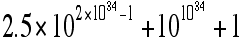8. 11

9. 182

10. 8,018,844,304 (eight billion eighteen million eight hundred forty-four thousand three hundred four), which is the square of 89,548.

11. 2,000,000,000,000,000,000,000,000,002,202,211,698,297,079,027,232,663,249,060,167,009 (two vigintillion two undecillion two hundred two decillion two hundred eleven nonillion six hundred ninety-eight octillion two hundred ninety-seven septillion seventy-nine sextillion twenty-seven quintillion two hundred thirty-two quadrillion six hundred sixty-three trillion two hundred forty-nine billion sixty million one hundred sixty-seven thousand nine), which is the square of 44,721,359,549,995,793,928,183,473,399,247.

12. "first";"second";"third"

13. e + (sinh 1)/4

14. I found a formula on an internet site,
http://mathforum.org/dr.math/faq/formulas/faq.analygeom_2.html#twotriangles ,
which aided me in determining the correct solution:
(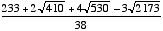,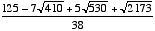).
Before finding the above formula I had gotten the solution simplified only to the point shown below after many attempts over many years:
Incenter is at (x0, y0), where

x0 =
3 +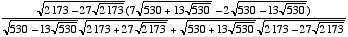,

and y0 =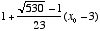.

15. a) 2

b)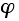(see #4 above)

c)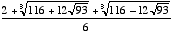d)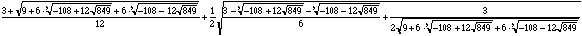16.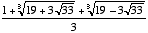17. a) I found expressions for 0, 1, 2, 4, 5, 6, 8, 10, 12, 16, 20, 22, 24, 26, 28, 44, 48, 60, 64, 96, 120, 256, 576, 720, 4096, 13824, 40320, 331776, 3628800, 16777216, 191102976, 479001600, 20922789888000, 281474976710656, 36520347436056576, 2432902008176640000, 1124000727777607680000, 25852016738884976640000, 155112100433309859840000, 248179360693295775744000, 310224200866619719680000, 620448401733239439359976, 620448401733239439359996, 620448401733239439359998, 620448401733239439360000, 620448401733239439360002, 620448401733239439360004, 620448401733239439360024, 1240896803466478878720000, 1551121004333098598400000, 2481793606932957757440000, 14890761641597746544640000, 403291461126605635584000000, 304888344611713860501504000000, 1333735776850284124449081472843776, 384956219213331276939737002152967117209600000000, 2658271574788448768043625811014615890319638528000000000, 12413915592536072670862289047373375038521486354677760000000000, 238845470948181951014676282087587661242764623832882511609856000000000000, 8320987112741390144276341183223364380754172606361245952449277696409600000000000000, 126886932185884164103433389335161480802865516174545192198801894375214704230400000000000000, and 148191290711022364575836328127140926575194970820976779184863158185815504490332160000000000000000.

b) I found expressions for 1/64, 1/60, 16/625, 1/24, 1/16, 8/125, 1/12, 1/10, 1/8, 4/25, 1/6, 1/5, 1/4, 2/5, 11/25, 1/2, 4/5, 8/5, 12/5, 5/2, 18/5, 22/5, 25/4, 48/5, 125/8, 118/5, 122/5, and 625/16.

c)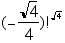would work.

d)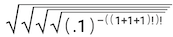18. If the radius of the circle is less than 1, there is no solution. Otherwise,
BP =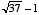.
[Update: It turns out there is a standard theorem that addresses this scenario, so evidently the problem is not so difficult as I had earlier believed.]

19. The mean is 333833.5 ; the median is 250500.5 .

20. 1: 1/60; 2: 1/12; 3: 1/60; 4: 1/12; 5: 1/60; 6: 1/12; 7: 1/60; 8: 1/60; 9: 1/60; 0: 13/20

21. 41,038

22. a) 1, 1, 1
xxxb) 1, 4, 4
xxxc) 16, 25, 36
xxxd) no solution (this is easily proven using the Pythagorean Theorem and Fermat's Last Theorem!)
xxxe) 1, 1, 1
xxxf) 9, 9, 16
xxxg) 16, 25, 36
xxxh) 64, 81, 100

23. Since the Hubble length is not defined with great precision, an exact answer is not possible. However, the answer would be roughly on the order of 10385.

24. I counted 11,148.

25.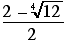26. You could say either "Bonjour!" (in French, the official language) or "Bara ala!" (in Sango, the national language) from your position at about 7.2ºN, 21.5ºE in Central African Republic. Your friend would likely reply "Asalaamu Aleikum" (in Arabic), or perhaps "Nabad" (in Somali), from his or her position at approximately 3.6ºN, 43.0ºE in Somalia.

27. 52

28. One such function would be: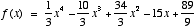,
which includes the points (1,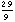), (2,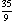), (3,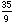), and (4,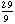); the tangents at these points enclose the square with vertices (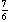,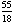), (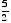,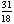), (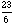,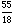), and (,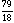), which has area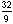.

29.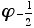(see #4 above)

30. a) 62
......b) Perimeter = 961; Angle measures (in radians): 1, 1, and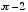; Sides: two each measuring 961/(2 + 2 cos 1), and one measuring (961 cos 1)/(1 + cos 1); Area: (923521 sin 2)/(12 + 16 cos 1 + 4 cos 2)

31.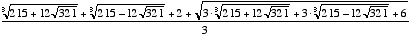32.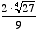33.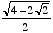34.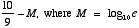35.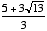36.   35

37. a)   45,360
b)   50,400 (which has 108 factors)

38.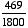(2345 out of 9000)

39.  a)  eight billion eighteen million eighteenth
b)  "Eighth" is the 1,001,001,001,001,001,001,001,002,001,002,001,001,001,001,001,001,001,002,001,001,002nd entry on the list.

40.  516

41.  2304

42. a)   276
b)   Each of two distinct triangles has the maximum area: one with perimeter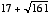and one with perimeter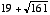.

43.  688

44.  9n-3(4n2-3n-1)

45.  1 — 0.9 ln 9! + 8.1 ln 10

46.  819199/819200 (which equals exactly 0.999998779296875)

47.  (sin 2°)/2

48. a)  2700
b)  7

49.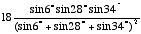50.  –3

51.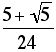,   which can also be expressed as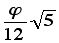,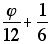,   or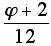52.  3, 5, 9, 15, 16. A very liberal interpretation of the conditions might also allow polygons of 48, 80, 144, and 240 sides. I choose not to consider these, since each would require a 180° angle to be regarded as an interior angle of a polygon.

53. a) 999,969
b) 28
c) 3

54. a) –2
b) 37,748,736

55. a) 0 < x < 10/3; 0 < y < 5; 10/3 < z < 10
b) 0 < x < 3; 1/2 < y < 5; 7/2 < z < 9
c) x = 1, 2, or 3; y = 1, 2, 3, or 4; z = 4, 5, 6, 7, or 8
d) x = 1, 2, or 3; y = 1, 2, 3, or 4; z = 4, 5, 6, 7, or 8

56.   9/65536

57. Either:
j = k = 1, 2, 3, or 4;
or:
j = 0, 1, 2, or 3; and k is any integer that exceeds j by at least 2.

58.   1061/1250, or .8488